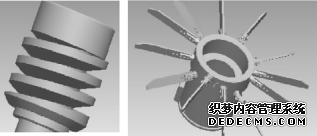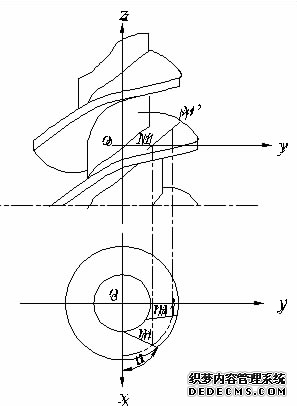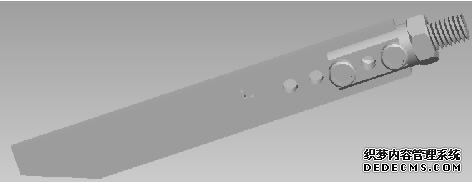# 搅拌机传动机构设计

1 概 述2 传动机构的设计与计算

2.1 螺旋杆的几何形状

T =(4/3)d j ～（10/3）d j .

2.2 螺旋型面的方程式

X=a cos( θ + τ )-b cos(m θ + τ ). Y=a sin( θ + τ )-b sin(m θ + τ ).

X=a cos(m θ + ∂ ＋ τ )-b cos(m θ + ∂ ＋ τ ). Y=a sin（θ + τ ＋ ∂ )-b sin(m θ + ∂ ＋ τ ).

Z =K δ .即取D a ＝110 mm. d j :D a = 图3 螺旋杆空间坐标系 3:5，可得d j =66 mm .

X=a cos(m θ + ∂ ＋ τ )-b cos(m θ + ∂ ＋ τ )

Y=a sin（θ + τ ＋ ∂ )-b sin(m θ + ∂ ＋ τ )

Z=K δ . X=0，Y=110.Z =24.445.

2.3 叶片的设计与计算

3 搅拌机的叶片设计4 结束语